Question

the image formed on a screen by a concave mirror of radius 20 cm is 3 times the size of the object. what is the distance of the screen from the mirror?​

1.Given that,

Radius of mirror = 20 cm

Size of image = 3 times of size of object

We need to calculate the distance of the screen from the mirror

Using formula of magnification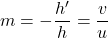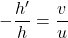Where, h’ = size of image

h = size of object

v = image distance from mirror

u = object distance from mirror

Put the value into the formula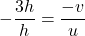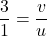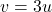The distance of image will three times of object distance.

Hence, The distance of the screen from the mirror is 3u.